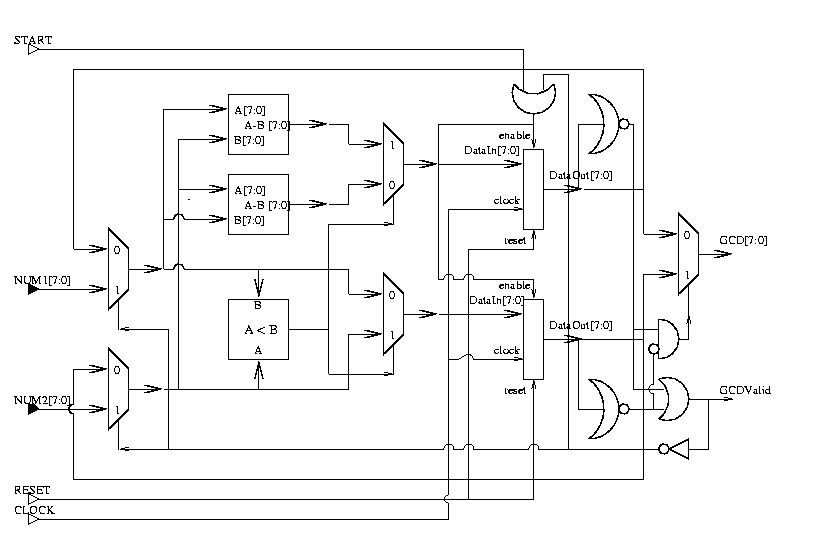﻿ 演算法筆記 - Algorithm

Algorithm

Algorithm

```          -----------------
input --->| computational |
| sequence      |---> output
-----------------
```

```GREATEST_COMMON_DIVISOR(a, b)
1   while a ≠ b do
2       if a > b then
3           a ← a - b
4       else
5           b ← b - a
6   return a
``````BUBBLESORT(A, n)                  | steps
1 for i ← 0 to n-1 do             | n
2     for j ← i to n-i-1 do       | n(n-1)/2
3         if A[j] < A[j+1] then   | n(n-1)/2
4             temp ← A[j]         | n(n-1)/2
5             A[j] ← A[j+1]       | n(n-1)/2
6             A[j+1] ← temp       | n(n-1)/2
```
```total = n + 5n(n-1)/2
= n + 2.5n² - 2.5n
= 2.5n² - 1.5n
= O(n²)
```

```               | time*       | space
---------------+-------------+--------
bubble sort    | O(n²)       | O(n)
insertion sort | O(n²)       | O(n)
merge sort     | O(n log(n)) | O(n)
quicksort      | O(n²)       | O(n)
heapsort       | O(n log(n)) | O(n)
counting sort  | O(n+r)      | O(n+r)

*worst case
```

Algorithm

```Algorithms
Robert Sedgewick and Kevin Wayne

Introduction to Algorithms
Thomas H. Cormen, Charles E. Leiserson, Ronald L. Rivest and Clifford Stein

Introduction to Algorithms: A Creative Approach
Udi Manber

Algorithm Design
Jon Kleinberg and Éva Tardos

Algorithm Design: Foundations, Analysis, and Internet Examples
Michael T. Goodrich and Roberto Tamassia

An Introduction to the Analysis of Algorithms
Robert Sedgewick and Philippe Flajolet

An Introduction to the Analysis of Algorithms
Michael Soltys
```

```Algorithms in a Nutshell: A Practical Guide
Gary Pollice, Stanley Selkow, George Heineman

The Algorithm Design Manual
Steven Skiena
```

```アルゴリズム図鑑 絵で見てわかる26のアルゴリズム

Grokking Algorithms: An illustrated guide for programmers and other curious people

```

```Out of their Minds: The Lives and Discoveries of 15 Great Computer Scientists

Nine Algorithms That Changed the Future: The Ingenious Ideas That Drive Today's Computers

Automate This: How Algorithms Came to Rule Our World

```

```Adam Blank

Jeff Erickson

Erik Demaine

```

```GeeksforGeeks

Algorithms Notes

Rosetta Code

LeetCode

```

```AMS Open Math Notes

Wolfram Math World

The On-Line Encyclopedia of Integer Sequences

```